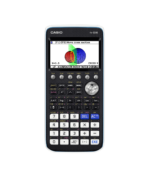Coordinate Geometry Archives - Casio Calculators

# Coordinate Geometry

Displaying Resources

FilterHow to Video
fx-CG50 A-Level
Tangents and Normals – Parametric Functions

How to sketch the tangent and normal to a curve in parametric form. Includes fin...How to Video
fx-CG50 A-Level IB
Tangents and Normals – Cartesian Functions

How to sketch the tangent and normal to a curve in Cartesian form. Includes find...How to Video
fx-CG50 A-Level
Simultaneous Equations – Solving Harder Equations Graphically

How to solve a simultaneous linear and quadratic equation in both x and y graphi...How to Video
fx-CG50 A-Level GCSE IB
Simultaneous Equations – Solving Graphically

How to solve two simultaneous linear, or linear and quadratic, equations using g...How to Video
fx-CG50 A-Level IB
Modulus Function – Graphs and Equations

How to plot the graph of a modulus function. Solve modulus equations both graphi...How to Video
fx-CG50 A-Level IB
Graphing – Types of Function

Plot graphs of parametric functions and where x = constant. Plot inequalities an...How to Video
fx-CG50 A-Level GCSE IB
Bivariate Data and Regression – Scatter Diagrams

How to plot a scatter diagram. Use linear regression to calculate regression sta...Teach Leaflet
fx-CG50 A-Level GCSE IB
Intersection of Line and Circle

This resource is an exercise for finding the intersection of a variety of lines ...Teach Leaflet
fx-CG50 A-Level GCSE

This activity consists of two sets of nine equation cards and a sheet of graphs...Teach Leaflet
fx-CG50 A-Level GCSE IB
Intersection of a Line and Curve

This activity asks learners to divide a set of 10 cards into four subsets accord...How to Leaflet
fx-CG50 Further Maths A-Level
Conic Graphs – Plot and Modify (Worksheet)

Worksheet to accompany the video on how to plot conic sections in rectangular, p...How to Leaflet
fx-CG50 Further Maths
Polar Functions – Graphing and Tables (Worksheet)

Worksheet to accompany the video on how to plot polar a graph and create the tab...How to Leaflet
fx-CG50 A-Level Further Maths
Conic Graph Quick Start fx-CG50

How to plot a conic function using built-in functions on the fx-CG50. Includes a...How to Leaflet
A-Level IB
Conics Quick Start fx-9860GII

How to plot a conic function using built-in functions on the fx-9860GII. Include...How to Video
fx-CG50 Further Maths
Polar Functions – Graphing and Tables

How to plot polar a graph and create the table of values. Restrict the graph for...How to Video
fx-CG50 Further Maths IB
3D Lines – Analysing

How to rotate the view, analyse the relationship between lines (skew, intersect,...How to Video
fx-CG50 Further Maths IB
3D Lines – Plotting

How to plot lines in 3D in Cartesian and vector forms, or through given points....